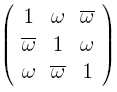occasional meanderings in physics' brave new world

## Monday, March 08, 2010

### M Theory Lesson 302

Assuming now that all matrix entries and scalars are complex numbers, let us consider a mass circulant $M$ in the formfor two parameters $r$ and $\theta$. Under the nonassociative exponential product, $M$ acts on the right of the democratic matrix as a scaling, as inwhereas its left action is of the formSo when $\lambda = r = 1$, the special scale free value of $\theta$ results in a familiar choice for $M$, namelywhere $\omega$ is a sixth root of unity. Note that a zero element in a left hand factor results in a totally zero matrix, so the nonassociative product has many zero divisors.L. Riofrio said...